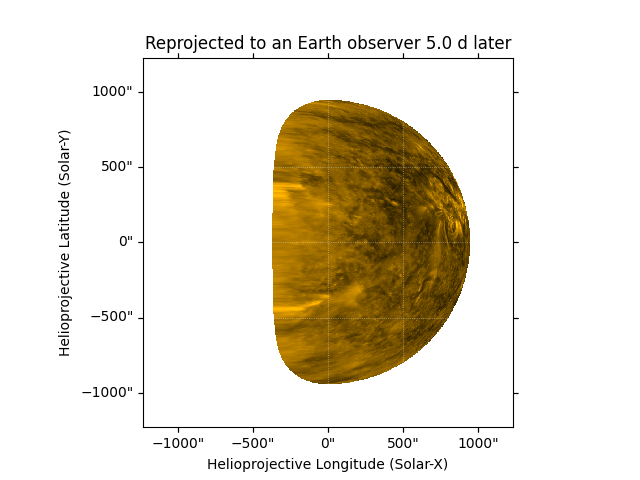# Differentially rotating a map#

How to apply differential rotation to a Map.

Note

This example requires reproject 0.6 or later to be installed.

The example uses the propagate_with_solar_surface() context manager to apply differential rotation during coordinate transformations.

import matplotlib.pyplot as plt

import astropy.units as u
from astropy.coordinates import SkyCoord
from astropy.wcs import WCS

import sunpy.map
from sunpy.coordinates import Helioprojective, propagate_with_solar_surface
from sunpy.data.sample import AIA_171_IMAGE


aiamap = sunpy.map.Map(AIA_171_IMAGE)
in_time = aiamap.date


Let’s define the output frame to be five days in the future for an observer at Earth (i.e., about five degrees offset in heliographic longitude compared to the location of AIA in the original observation).

out_time = in_time + 5*u.day
out_frame = Helioprojective(observer='earth', obstime=out_time,
rsun=aiamap.coordinate_frame.rsun)


Construct a WCS object for the output map. If one has an actual Map object at the desired output time (e.g., the actual AIA observation at the output time), one can use the WCS object from that Map object (e.g., mymap.wcs) instead of constructing a custom WCS.

out_center = SkyCoord(0*u.arcsec, 0*u.arcsec, frame=out_frame)
out_center,
scale=u.Quantity(aiamap.scale))


Reproject the map from the input frame to the output frame. We use the propagate_with_solar_surface() context manager so that coordinates are treated as points that evolve in time with the rotation of the solar surface rather than as inertial points in space.

with propagate_with_solar_surface():
out_warp = aiamap.reproject_to(out_wcs)


Let’s plot the differentially rotated Map next to the original Map.

fig = plt.figure(figsize=(12, 4))

aiamap.plot(axes=ax1, vmin=0, vmax=20000, title='Original map')
plt.colorbar()